# Chapter 27 Quantum Physics Need for Quantum Physics

• Slides: 44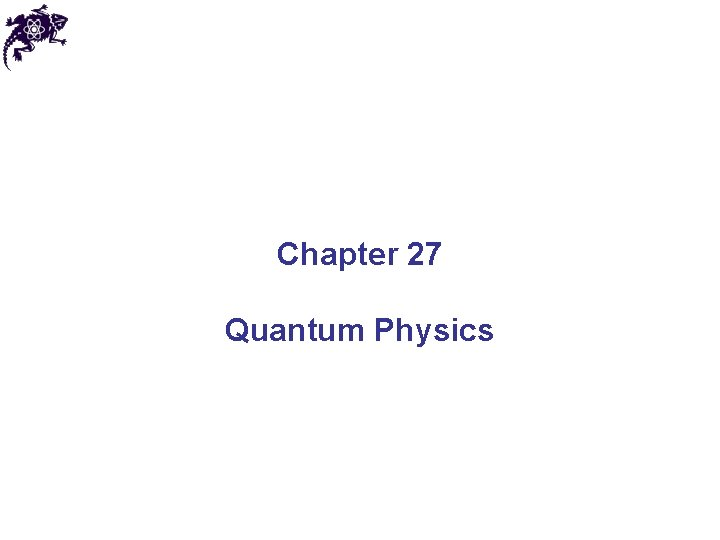Chapter 27 Quantum Physics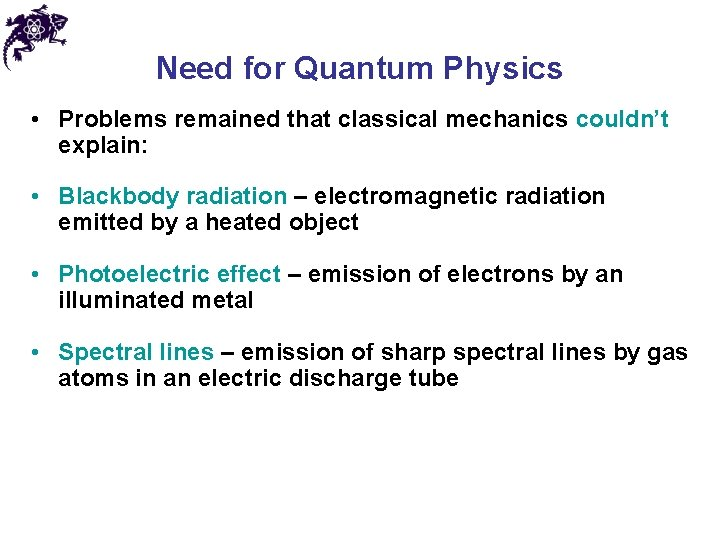Need for Quantum Physics • Problems remained that classical mechanics couldn’t explain: • Blackbody radiation – electromagnetic radiation emitted by a heated object • Photoelectric effect – emission of electrons by an illuminated metal • Spectral lines – emission of sharp spectral lines by gas atoms in an electric discharge tube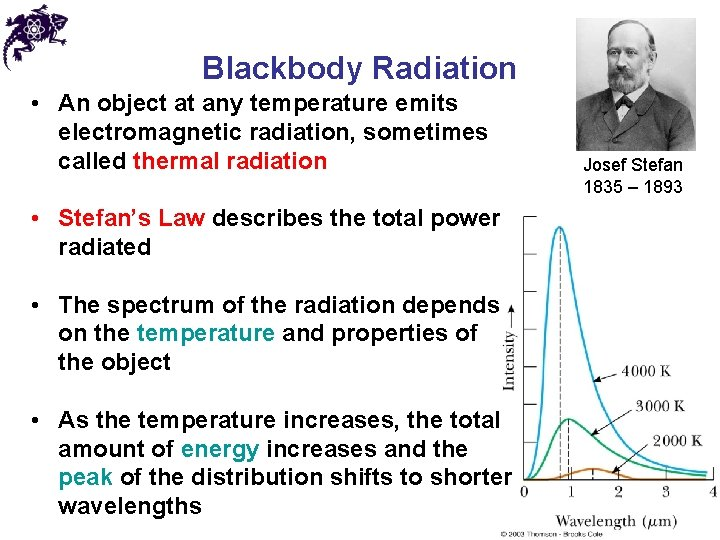Blackbody Radiation • An object at any temperature emits electromagnetic radiation, sometimes called thermal radiation • Stefan’s Law describes the total power radiated • The spectrum of the radiation depends on the temperature and properties of the object • As the temperature increases, the total amount of energy increases and the peak of the distribution shifts to shorter wavelengths Josef Stefan 1835 – 1893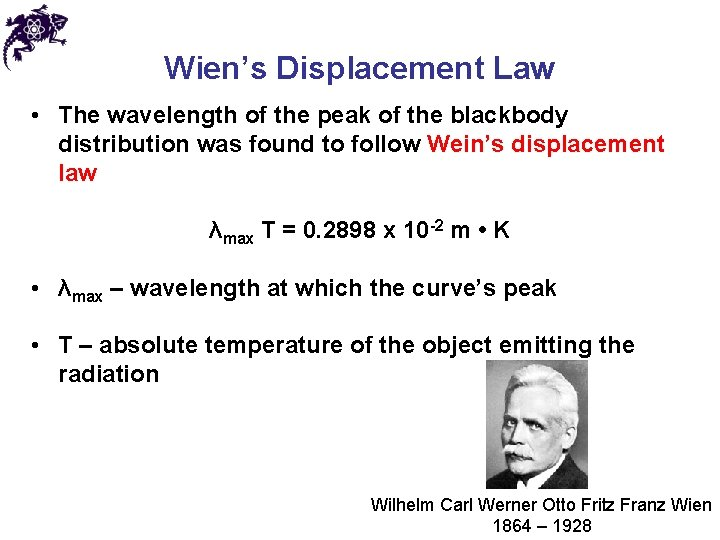Wien’s Displacement Law • The wavelength of the peak of the blackbody distribution was found to follow Wein’s displacement law λmax T = 0. 2898 x 10 -2 m • K • λmax – wavelength at which the curve’s peak • T – absolute temperature of the object emitting the radiation Wilhelm Carl Werner Otto Fritz Franz Wien 1864 – 1928The Ultraviolet Catastrophe • Classical theory did not match the experimental data • At long wavelengths, the match is good • At short wavelengths, classical theory predicted infinite energy • At short wavelengths, experiment showed no or little energy • This contradiction is called the ultraviolet catastrophe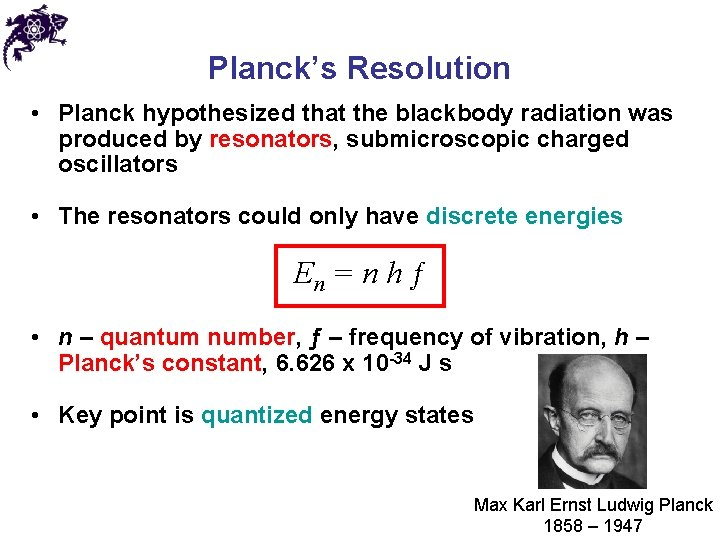Planck’s Resolution • Planck hypothesized that the blackbody radiation was produced by resonators, submicroscopic charged oscillators • The resonators could only have discrete energies En = n h ƒ • n – quantum number, ƒ – frequency of vibration, h – Planck’s constant, 6. 626 x 10 -34 J s • Key point is quantized energy states Max Karl Ernst Ludwig Planck 1858 – 1947Photoelectric Effect • Photoelectric effect (first discovered by Hertz): when light is incident on certain metallic surfaces, electrons are emitted from the surface – photoelectrons • The successful explanation of the effect was given by Einstein • Electrons collected at C (maintained at a positive potential) and passing through the ammeter are a current in the circuit Albert Einstein 1879 – 1955Photoelectric Effect • The current increases with intensity, but reaches a saturation level for large DV’s • No current flows for voltages less than or equal to –DVs, the stopping potential, independent of the radiation intensity • The maximum kinetic energy of the photoelectrons is related to the stopping potential: KEmax = e DVsFeatures Not Explained by Classical Physics • No electrons are emitted if the incident light frequency is below some cutoff frequency that is characteristic of the material being illuminated • The maximum kinetic energy of the photoelectrons is independent of the light intensity • The maximum kinetic energy of the photoelectrons increases with increasing light frequency • Electrons are emitted from the surface almost instantaneously, even at low intensities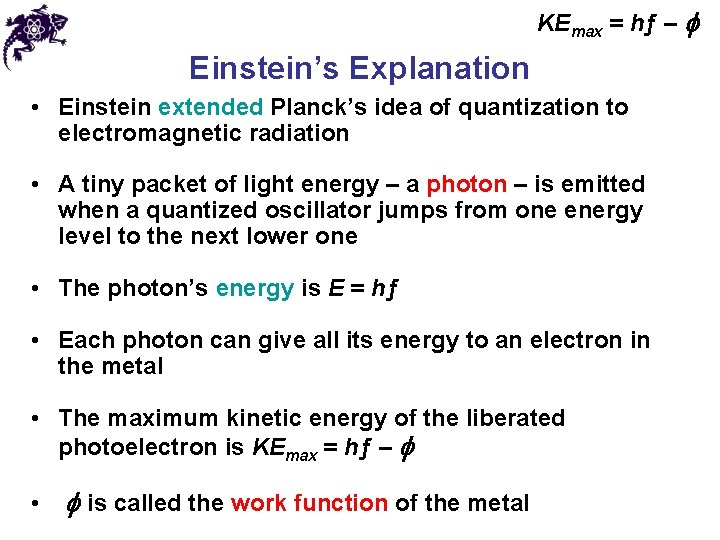KEmax = hƒ – f Einstein’s Explanation • Einstein extended Planck’s idea of quantization to electromagnetic radiation • A tiny packet of light energy – a photon – is emitted when a quantized oscillator jumps from one energy level to the next lower one • The photon’s energy is E = hƒ • Each photon can give all its energy to an electron in the metal • The maximum kinetic energy of the liberated photoelectron is KEmax = hƒ – f • f is called the work function of the metalKEmax = hƒ – f Einstein’s Explanation • The effect is not observed below a certain cutoff frequency since the photon energy must be greater than or equal to the work function • Without this, electrons are not emitted, regardless of the intensity of the light • The maximum KE depends only on the frequency and the work function, not on the intensity • The maximum KE increases with increasing frequency • The effect is instantaneous since there is a one-to-one interaction between the photon and the electronKEmax = hƒ – f Verification of Einstein’s Theory • Experimental observations of a linear relationship between KE and frequency confirm Einstein’s theory • The x-intercept is the cutoff frequency • The cutoff wavelength is related to the work function • Wavelengths greater than l. C incident on a material with a work function f don’t result in the emission of photoelectrons c = λƒ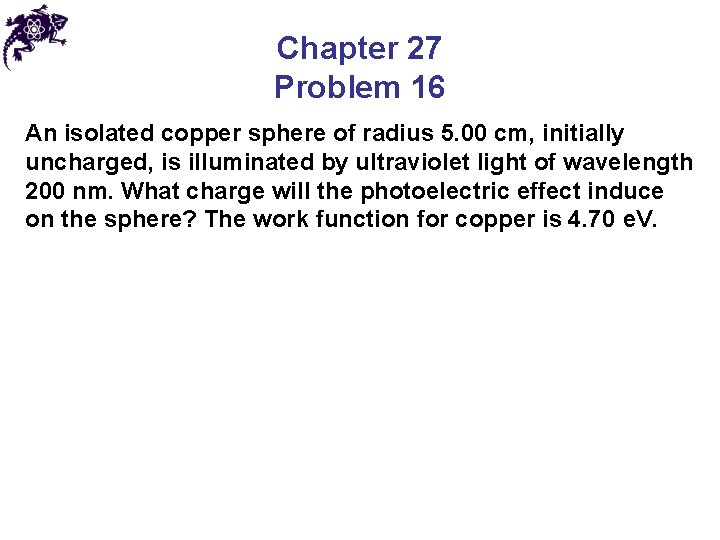Chapter 27 Problem 16 An isolated copper sphere of radius 5. 00 cm, initially uncharged, is illuminated by ultraviolet light of wavelength 200 nm. What charge will the photoelectric effect induce on the sphere? The work function for copper is 4. 70 e. V.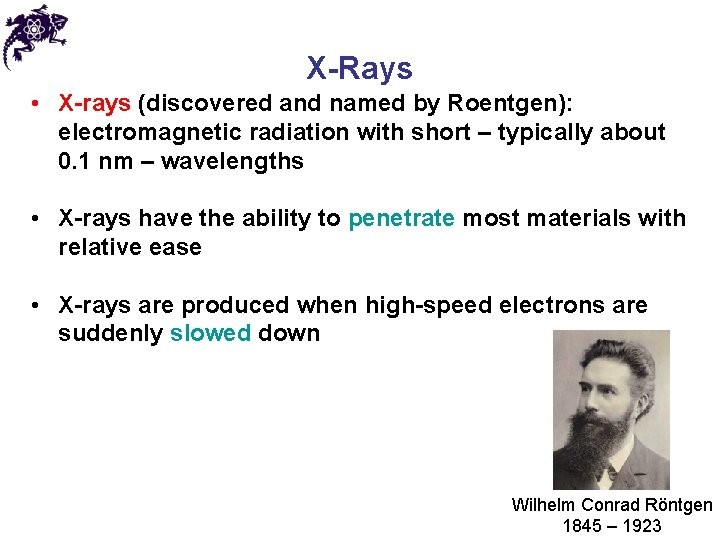X-Rays • X-rays (discovered and named by Roentgen): electromagnetic radiation with short – typically about 0. 1 nm – wavelengths • X-rays have the ability to penetrate most materials with relative ease • X-rays are produced when high-speed electrons are suddenly slowed down Wilhelm Conrad Röntgen 1845 – 1923Production of X-rays • X-rays can be produced by electrons striking a metal target • A current in the filament causes electrons to be emitted • These freed electrons are accelerated toward a dense metal target (the target is held at a higher potential than the filament)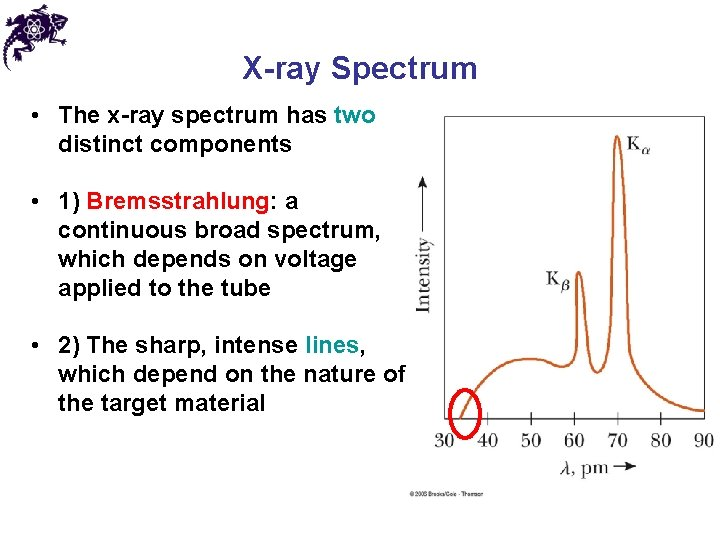X-ray Spectrum • The x-ray spectrum has two distinct components • 1) Bremsstrahlung: a continuous broad spectrum, which depends on voltage applied to the tube • 2) The sharp, intense lines, which depend on the nature of the target material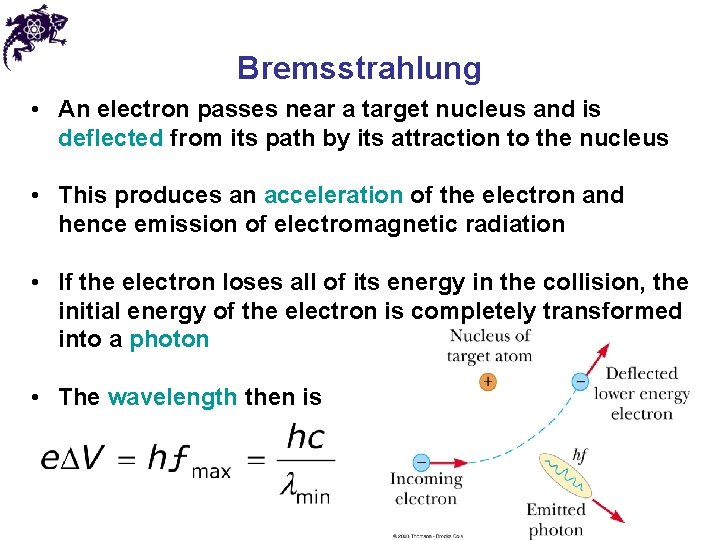Bremsstrahlung • An electron passes near a target nucleus and is deflected from its path by its attraction to the nucleus • This produces an acceleration of the electron and hence emission of electromagnetic radiation • If the electron loses all of its energy in the collision, the initial energy of the electron is completely transformed into a photon • The wavelength then is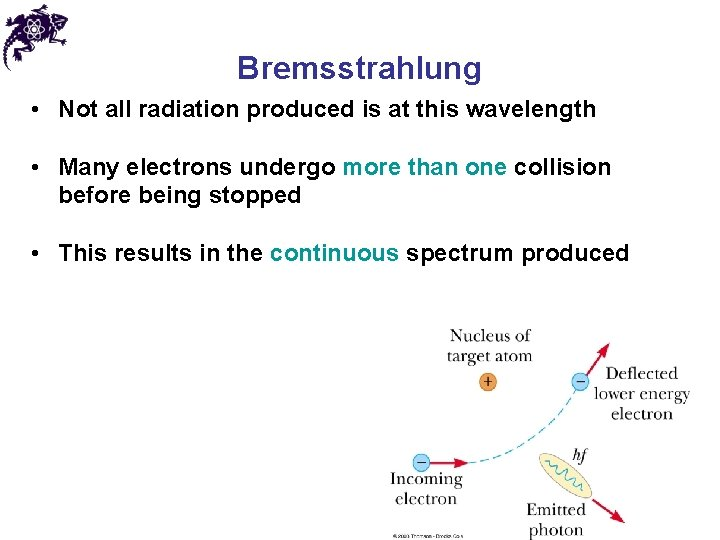Bremsstrahlung • Not all radiation produced is at this wavelength • Many electrons undergo more than one collision before being stopped • This results in the continuous spectrum produced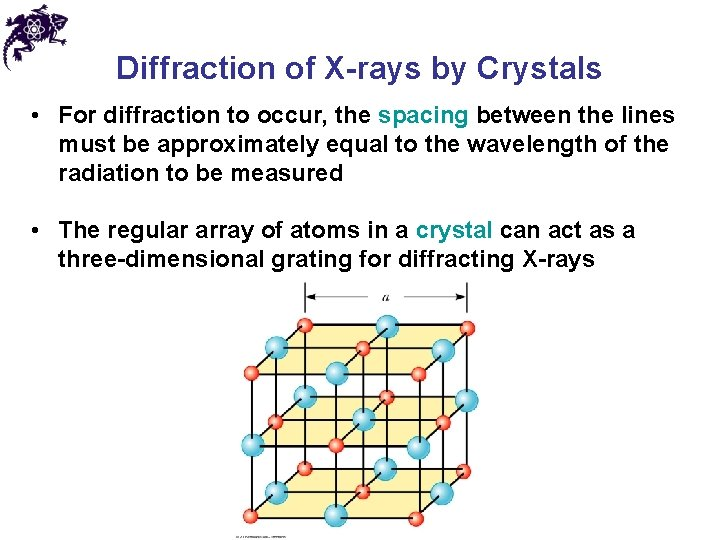Diffraction of X-rays by Crystals • For diffraction to occur, the spacing between the lines must be approximately equal to the wavelength of the radiation to be measured • The regular array of atoms in a crystal can act as a three-dimensional grating for diffracting X-rays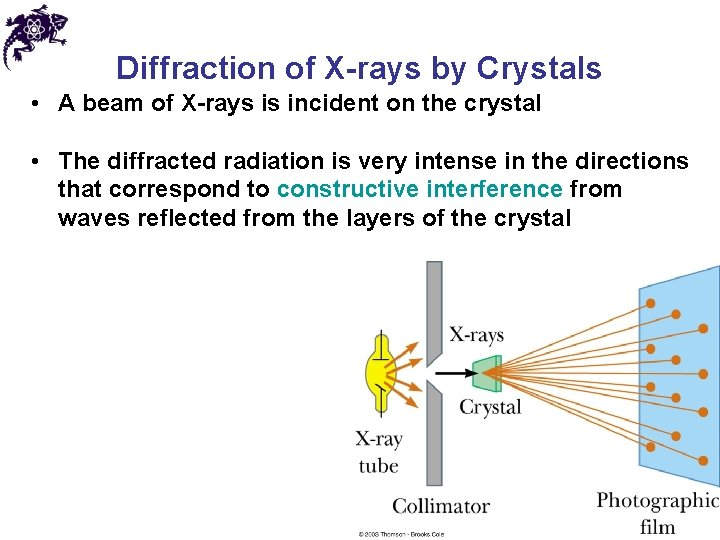Diffraction of X-rays by Crystals • A beam of X-rays is incident on the crystal • The diffracted radiation is very intense in the directions that correspond to constructive interference from waves reflected from the layers of the crystal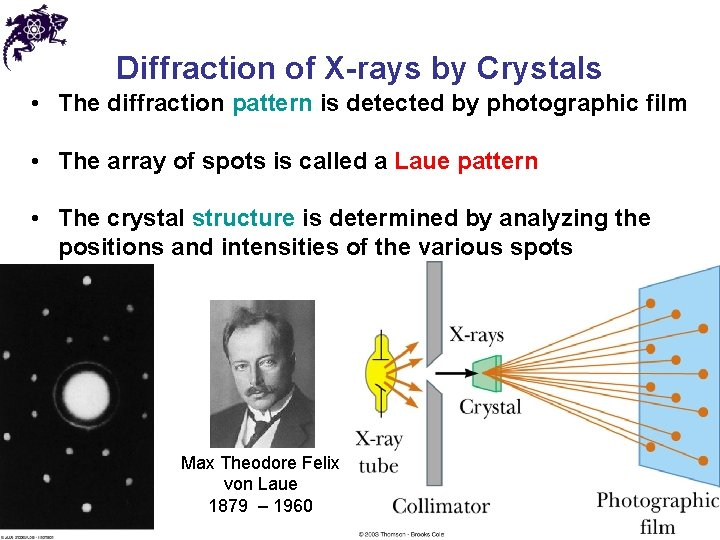Diffraction of X-rays by Crystals • The diffraction pattern is detected by photographic film • The array of spots is called a Laue pattern • The crystal structure is determined by analyzing the positions and intensities of the various spots Max Theodore Felix von Laue 1879 – 1960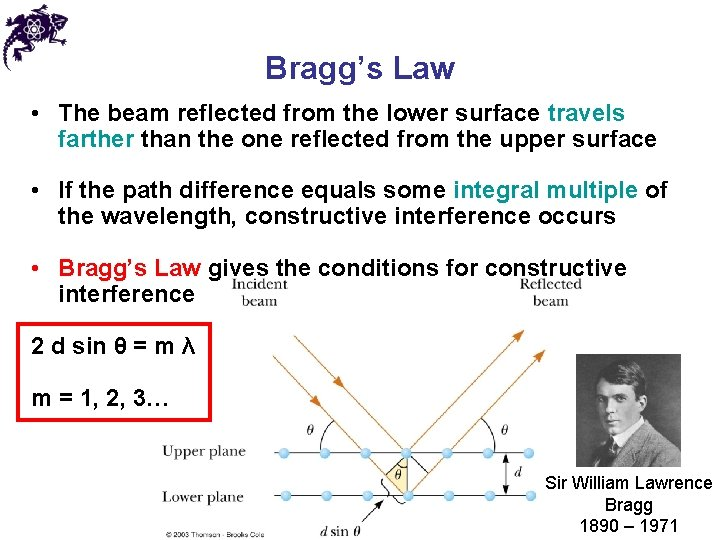Bragg’s Law • The beam reflected from the lower surface travels farther than the one reflected from the upper surface • If the path difference equals some integral multiple of the wavelength, constructive interference occurs • Bragg’s Law gives the conditions for constructive interference 2 d sin θ = m λ m = 1, 2, 3… Sir William Lawrence Bragg 1890 – 1971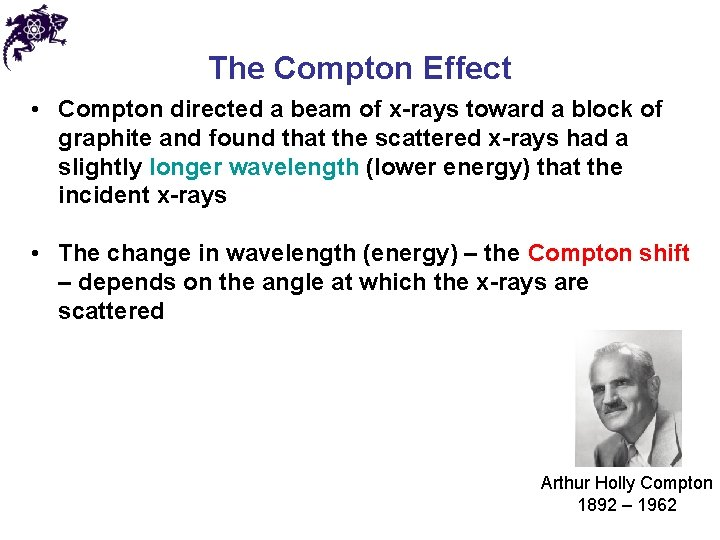The Compton Effect • Compton directed a beam of x-rays toward a block of graphite and found that the scattered x-rays had a slightly longer wavelength (lower energy) that the incident x-rays • The change in wavelength (energy) – the Compton shift – depends on the angle at which the x-rays are scattered Arthur Holly Compton 1892 – 1962The Compton Effect • Compton assumed the photons acted like other particles in collisions with electrons • Energy and momentum were conserved • The shift in wavelength is given by Arthur Holly Compton 1892 – 1962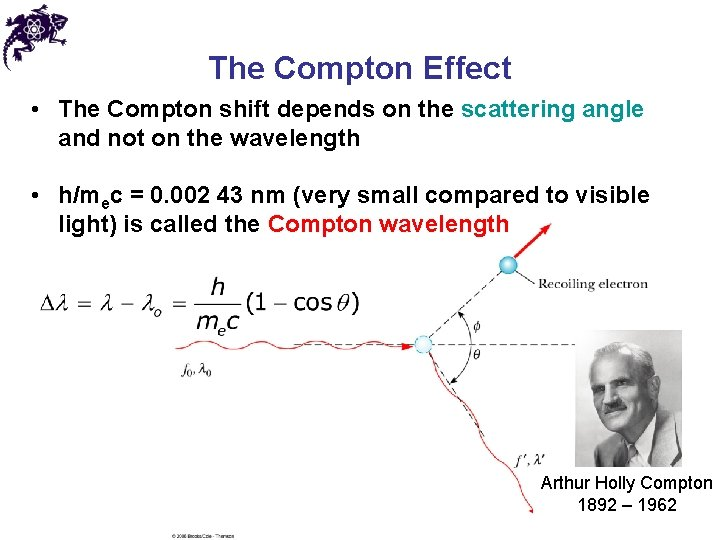The Compton Effect • The Compton shift depends on the scattering angle and not on the wavelength • h/mec = 0. 002 43 nm (very small compared to visible light) is called the Compton wavelength Arthur Holly Compton 1892 – 1962Chapter 27 Problem 33 A 0. 45 -nm x-ray photon is deflected through a 23° angle after scattering from a free electron. (a) What is the kinetic energy of the recoiling electron? (b) What is its speed?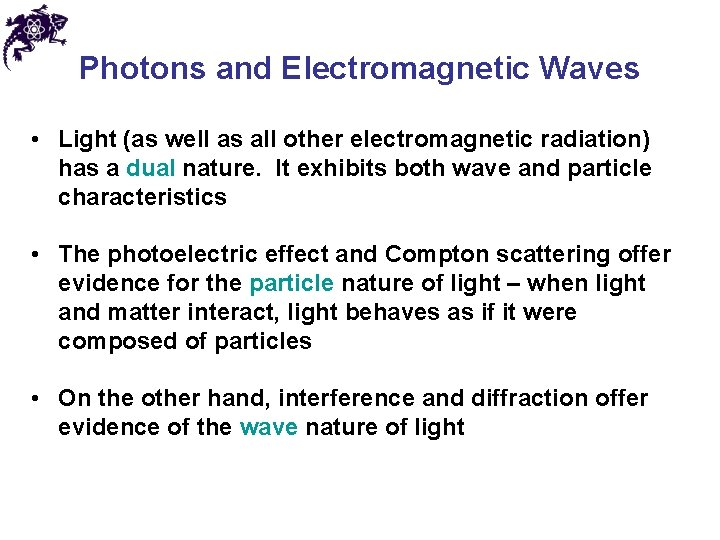Photons and Electromagnetic Waves • Light (as well as all other electromagnetic radiation) has a dual nature. It exhibits both wave and particle characteristics • The photoelectric effect and Compton scattering offer evidence for the particle nature of light – when light and matter interact, light behaves as if it were composed of particles • On the other hand, interference and diffraction offer evidence of the wave nature of lightWave Properties of Particles • In 1924, Louis de Broglie postulated that because photons have wave and particle characteristics, perhaps all forms of matter have both properties • Furthermore, the frequency and wavelength of matter waves can be determined • The de Broglie wavelength of a particle is • The frequency of matter waves is Louis de Broglie 1892 – 1987Wave Properties of Particles • The de Broglie equations show the dual nature of matter • Each contains matter concepts (energy and momentum) and wave concepts (wavelength and frequency) • The de Broglie wavelength of a particle is • The frequency of matter waves is Louis de Broglie 1892 – 1987The Davisson-Germer Experiment • Davisson and Germer scattered low-energy electrons from a nickel target and followed this with extensive diffraction measurements from various materials • The wavelength of the electrons calculated from the diffraction data agreed with the expected de Broglie wavelength • This confirmed the wave nature of electrons • Other experimenters confirmed the wave nature of other particles Clinton Joseph Davisson (1881 – 1958) and Lester Halbert Germer (1896 – 1971)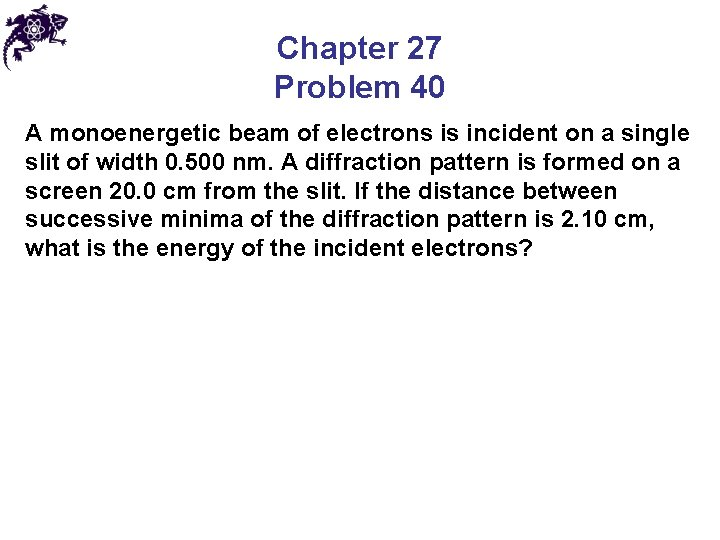Chapter 27 Problem 40 A monoenergetic beam of electrons is incident on a single slit of width 0. 500 nm. A diffraction pattern is formed on a screen 20. 0 cm from the slit. If the distance between successive minima of the diffraction pattern is 2. 10 cm, what is the energy of the incident electrons?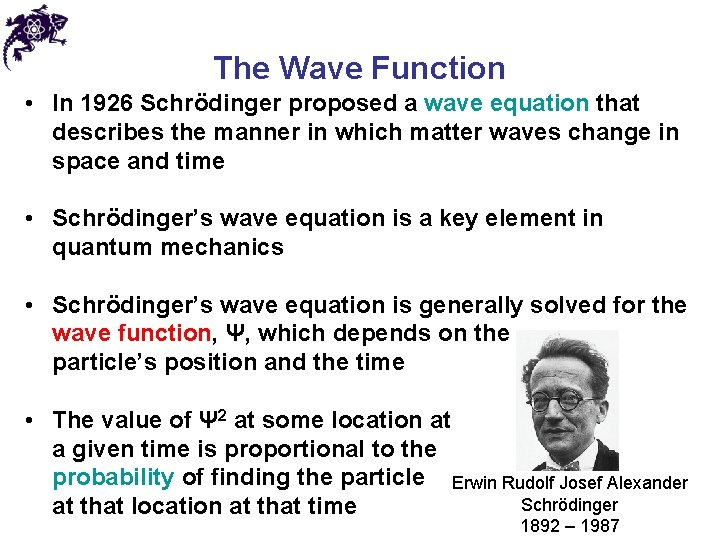The Wave Function • In 1926 Schrödinger proposed a wave equation that describes the manner in which matter waves change in space and time • Schrödinger’s wave equation is a key element in quantum mechanics • Schrödinger’s wave equation is generally solved for the wave function, Ψ, which depends on the particle’s position and the time • The value of Ψ 2 at some location at a given time is proportional to the probability of finding the particle Erwin Rudolf Josef Alexander Schrödinger at that location at that time 1892 – 1987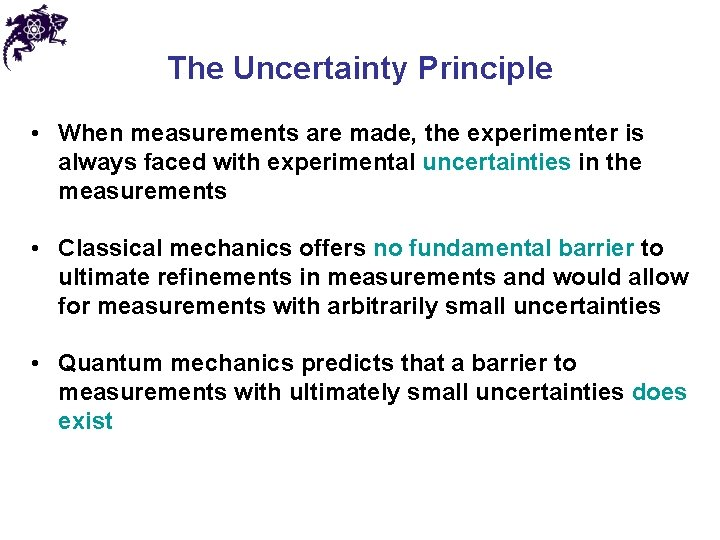The Uncertainty Principle • When measurements are made, the experimenter is always faced with experimental uncertainties in the measurements • Classical mechanics offers no fundamental barrier to ultimate refinements in measurements and would allow for measurements with arbitrarily small uncertainties • Quantum mechanics predicts that a barrier to measurements with ultimately small uncertainties does exist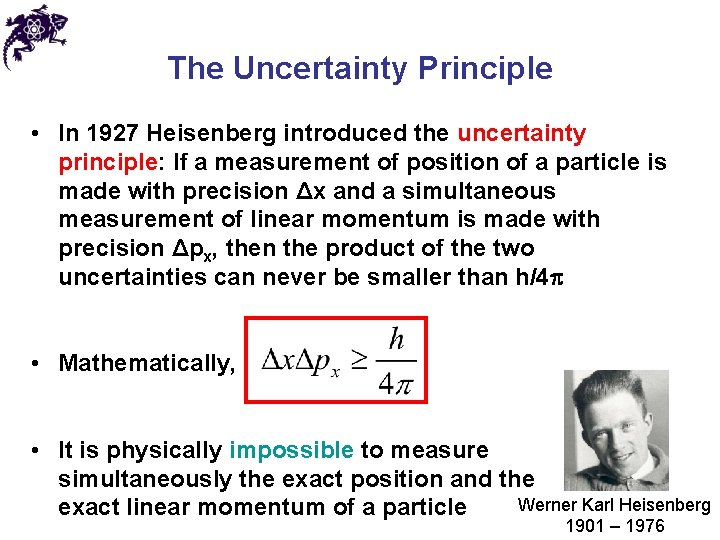The Uncertainty Principle • In 1927 Heisenberg introduced the uncertainty principle: If a measurement of position of a particle is made with precision Δx and a simultaneous measurement of linear momentum is made with precision Δpx, then the product of the two uncertainties can never be smaller than h/4 • Mathematically, • It is physically impossible to measure simultaneously the exact position and the Werner Karl Heisenberg exact linear momentum of a particle 1901 – 1976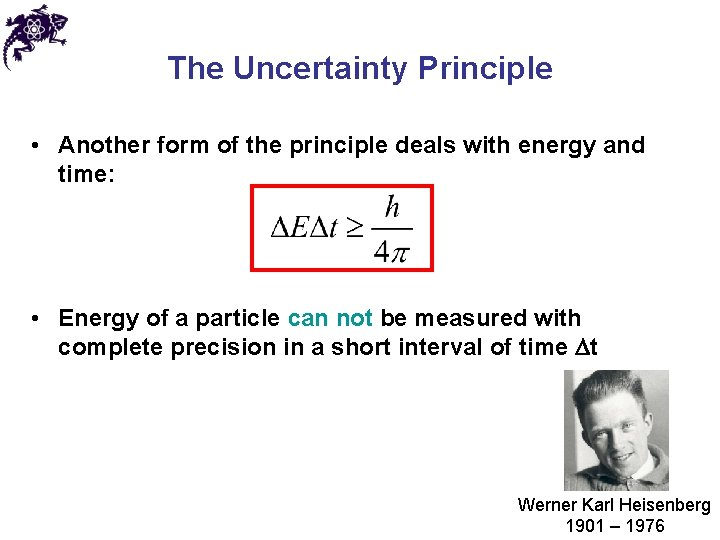The Uncertainty Principle • Another form of the principle deals with energy and time: • Energy of a particle can not be measured with complete precision in a short interval of time Dt Werner Karl Heisenberg 1901 – 1976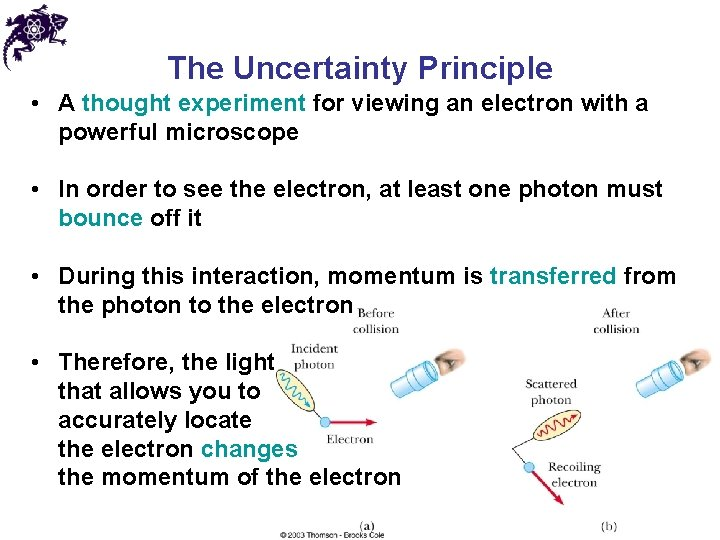The Uncertainty Principle • A thought experiment for viewing an electron with a powerful microscope • In order to see the electron, at least one photon must bounce off it • During this interaction, momentum is transferred from the photon to the electron • Therefore, the light that allows you to accurately locate the electron changes the momentum of the electron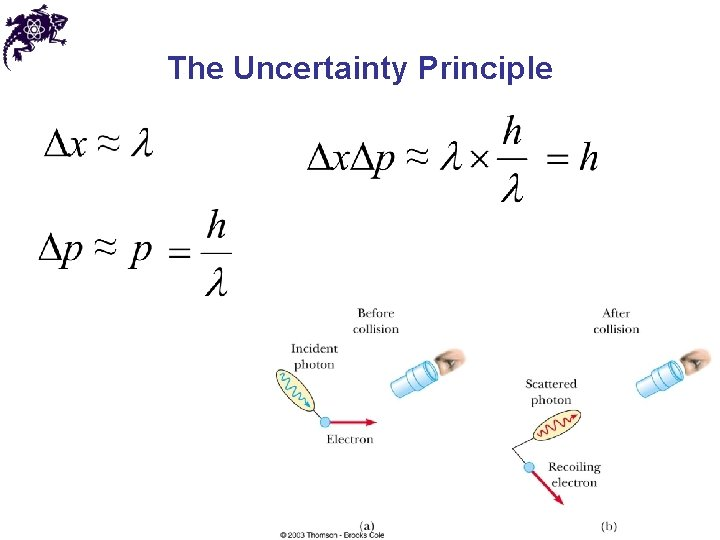The Uncertainty PrincipleChapter 27 Problem 46 (a) Show that the kinetic energy of a nonrelativistic particle can be written in terms of its momentum as KE = p 2/2 m. (b) Use the results of (a) to find the minimum kinetic energy of a proton confined within a nucleus having a diameter of 1. 0 × 10− 15 m.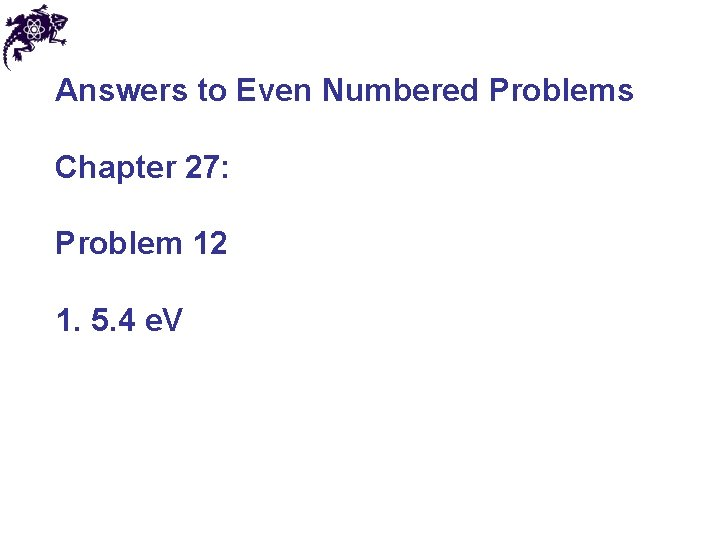Answers to Even Numbered Problems Chapter 27: Problem 12 1. 5. 4 e. V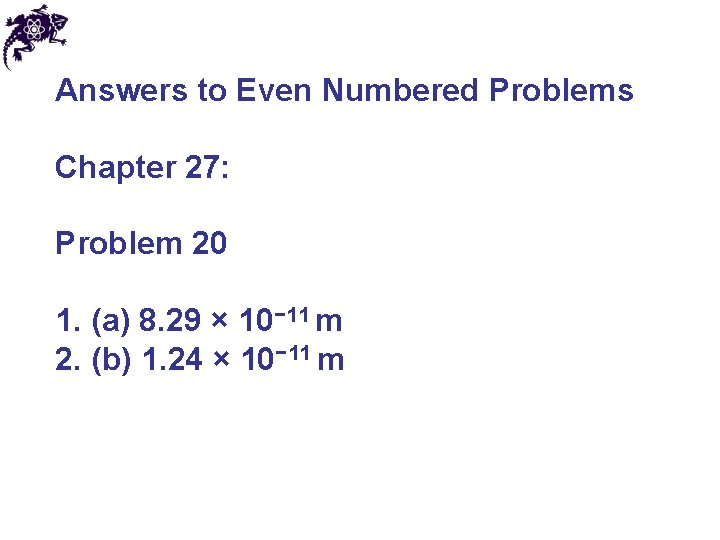Answers to Even Numbered Problems Chapter 27: Problem 20 1. (a) 8. 29 × 10− 11 m 2. (b) 1. 24 × 10− 11 mAnswers to Even Numbered Problems Chapter 27: Problem 24 1. 6. 7°Answers to Even Numbered Problems Chapter 27: Problem 28 1. 1. 8 ke. V, 9. 7 × 10− 25 kg⋅m/sAnswers to Even Numbered Problems Chapter 27: Problem 34 (a) 1. 98 × 10− 11 m (b) 1. 98 × 10− 14 mAnswers to Even Numbered Problems Chapter 27: Problem 52 1. 191 Me. V